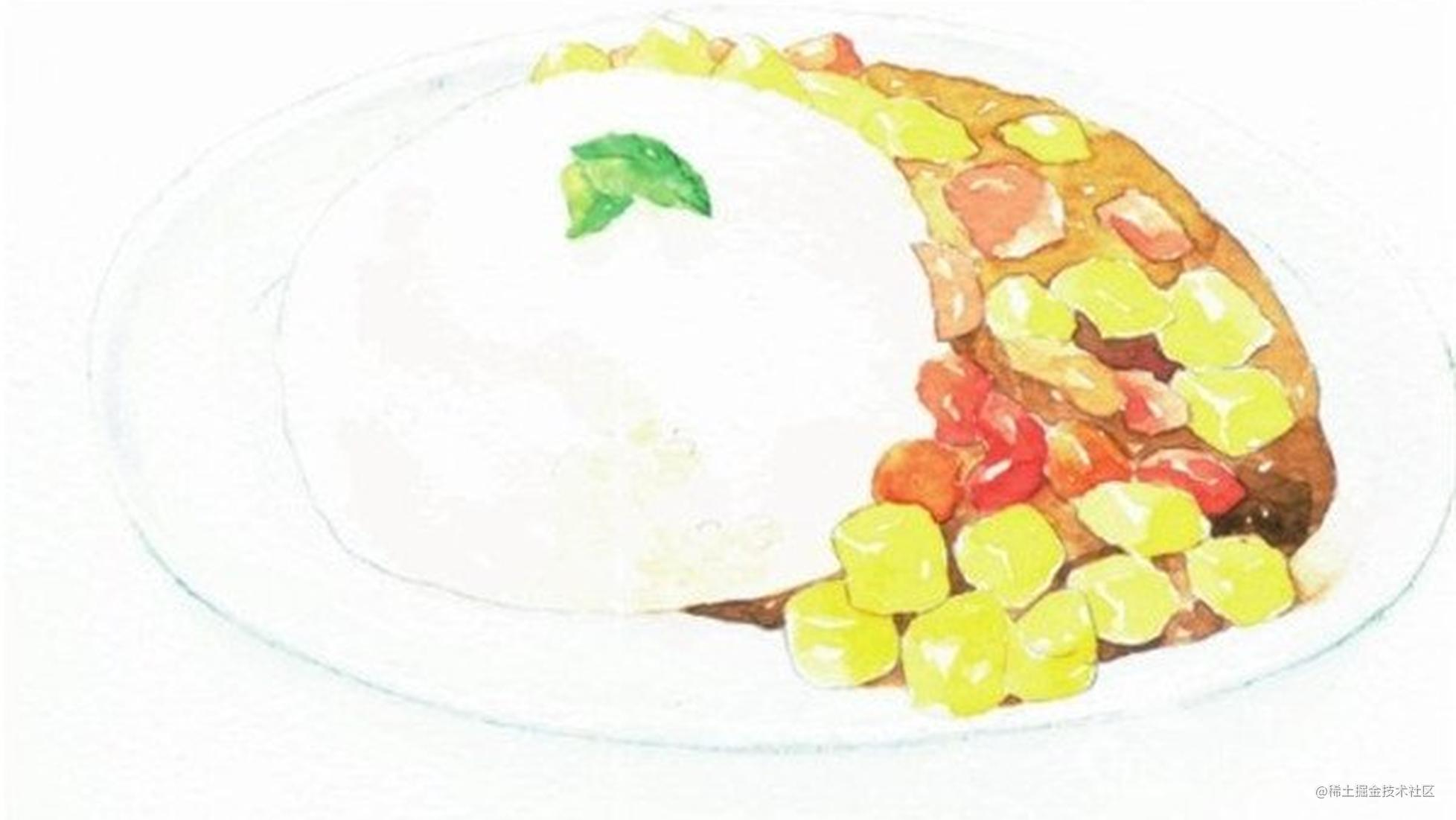# 当我们谈Currying时，我们在谈些什么

f(A)->B

f(X1, X2, ..., Xn)->->g(X1)(X2)...(Xn)

``````add(1, 2, 3); // 6

``````let sum = 0;
sum += Array.from(arguments).reduce((x, y) => x + y);
add.toString = () => sum; // console.log时实际调用的是Function.toString()
}

``````function add() {
// 第一次调用时，就要对参数求和并保存到total中
let total = Array.from(arguments).reduce((x, y) => x + y);
// 利用闭包特性，保存total
const _add = function () { // 注意此处不能写成箭头函数
total += Array.from(arguments).reduce((x, y) => x + y);
}
}

—— 维基百科

``````function add() {
// 把参数保存到一个数组里
const arr = Array.from(arguments);
// 利用闭包特性，保存total
const _add = function () { // 注意此处不能写成箭头函数
arr.push(...Array.from(arguments));
}
_add.toString = () => Array.from(arr).reduce((x, y) => x + y);
}

``````function fnToCurry(fn) {
const curry = function () {
// 第一次调用时，就把参数保存到数组里
const arr = Array.from(arguments);
// 利用闭包特性，保存total
const _fn = function () { // 注意此处不能写成箭头函数
arr.push(...Array.from(arguments));
return _fn;
}
_fn.toString = () => fn(...arr);
return _fn;
}
return curry; // 最后返回柯里化后的函数
}

``````function f1() {
return Array.from(arguments).reduce((x, y) => x + y);
}
// 将f1柯里化
const f2 = fnToCurry(f1);
console.log(f2(1, 2, 3)); // 6
console.log(f2(1)(2)(3)); // 6

``````function fnToDelayCurry(fn) {
const curry = function () {
const arr = Array.from(arguments)
// 利用闭包特性，保存total
const _fn = function () { // 注意此处不能写成箭头函数
// 当输入的参数中有exec标志时，立即执行
// 主要就是在这里去对传入的参数作判断，来判断是否执行
// 这里包含exec的参数不会被保存到arr中，若要保存exec，则把else中的push挪到外层即可
if (Array.from(arguments).indexOf("exec") > -1) {
_fn.toString = () => fn(...arr);
} else {
_fn.toString = Function.toString;
arr.push(...Array.from(arguments));
}
return _fn;
}
return _fn;
}
return curry;
}

``````function delayFn() {
const args = Array.from(arguments);
return args.join("~");
}
console.log(fnToDelayCurry(delayFn)(1)(2)(3)(4, 5)); // 这里打印的是函数_fn
console.log(fnToDelayCurry(delayFn)(1)(2)(3)(4, 5)("exec")); // 1~2~3~4~5

1. 柯里化[wiki]：en.wikipedia.org/wiki/Curryi…
2. 闭包：developer.mozilla.org/zh-CN/docs/…
3. λ演算-函数式语言的起源：zhuanlan.zhihu.com/p/164700404

### 往期文章

ES2021新特性

requestAnimationFrame